# Clustered Columnstore Indexes – part 49 (“Data Types & Predicate Pushdown”)

Continuation from the previous 48 parts, starting from https://www.nikoport.com/2013/07/05/clustered-columnstore-indexes-part-1-intro/

Note: Updated on 05th of March 2015 with information on SQL Server 2012.

Having blogged a couple of times on the matters of Segment Elimination (“Data Loading for Better Segment Elimination“, “Deleted Segments Elimination” ), I decided to go 1 step deeper in this direction and see which data types support segment elimination and which not.

For this purpose I will re-create my table MaxDataTable will all supported Data Types and then will run test queries on it, determining if Predicate Pushdown is taking place on the Data Type in question:

```create table dbo.MaxDataTable(
c1 bigint identity(1,1),
c2 numeric (36,3),
c3 bit,
c4 smallint,
c5 decimal (18,3),
c6 smallmoney,
c7 int,
c8 tinyint,
c9 money,
c10 float(24),
c11 real,
c12 date,
c13 datetimeoffset,
c14 datetime2 (7),
c15 smalldatetime,
c16 datetime,
c17 time (7),
c18 char(100),
c19 varchar(100),
c20 nchar(100),
c21 nvarchar(100),
c22 binary(16),
c23 varbinary(16),
c24 uniqueidentifier,
);
```

Next step is to load 2.5 Million Rows, so I would have some data to play with:

```set nocount on

-- Insert 2.500.000 rows in order to get a couple Row Groups
declare @i as int;
declare @max as int;
select @max = isnull(max(C1),0) from dbo.MaxDataTable;
set @i = 1;

begin tran
while @i <= 2500000
begin
insert into dbo.MaxDataTable
default values

set @i = @i + 1;

if( @i % 1000000 = 0 )
begin
commit;
checkpoint
begin tran
end
end;
commit;

checkpoint
```

Now I am ready to update the table with some random data, and notice that for numerical values I am putting lower values (150 and below) into the first segment (1045678 rows):

```checkpoint

-- Update our table with some pretty random data

;with updTable as
(
select *
, row_number() over(partition by C1 order by C1) as rnk
from dbo.MaxDataTable
)
update updTable
set C2 = ABS(CHECKSUM(NewId())) % 150.0,
C3 = 0, --ABS(CHECKSUM(NewId())) % 1,
C4 = cast(ABS(CHECKSUM(NewId())) % 150 as smallint),
C5 = ABS(CHECKSUM(NewId())) % 150.0,
C6 = cast(ABS(CHECKSUM(NewId())) % 150.0 as smallmoney),
C7 = ABS(CHECKSUM(NewId())) % 150,
C8 = ABS(CHECKSUM(NewId())) % 150,
C9 = ABS(CHECKSUM(NewId())) % 150.0,
C10 = ABS(CHECKSUM(NewId())) % 150.0,
C11 = ABS(CHECKSUM(NewId())) % 150.0,
C13 = ToDateTimeOffset( DATEADD(day, (ABS(CHECKSUM(NEWID())) % 150), 0), '+01:00'),
C14 = DATEADD(day, (ABS(CHECKSUM(NEWID())) % 150), 0),
C15 = DATEADD(day, (ABS(CHECKSUM(NEWID())) % 150), 0),
C16 = DATEADD(day, (ABS(CHECKSUM(NEWID())) % 150), 0),
C18 = cast( ABS(CHECKSUM(NewId())) % 150.0 as char(25)),
C19 = cast( ABS(CHECKSUM(NewId())) % 150.0 as varchar(25)),
C20 = cast( ABS(CHECKSUM(NewId())) % 150.0 as nchar(25)),
C21 = cast( ABS(CHECKSUM(NewId())) % 150.0 as nvarchar(25)),
C22 = convert(binary(16), newid()),
C23 = convert(binary(16), newid()),
C24 = NewId()
where c1 <= 1048576;

-- Update our table with some pretty random data
;with updTable as
(
select *
, row_number() over(partition by C1 order by C1) as rnk
from dbo.MaxDataTable
)
update updTable
set C2 = ABS(CHECKSUM(NewId())) % 142359.0,
C3 = 1,
C4 = cast(ABS(CHECKSUM(NewId())) % 10000 as smallint),
C5 = ABS(CHECKSUM(NewId())) % 242359.0,
C6 = ABS(CHECKSUM(NewId())) % 214748.0,
C7 = ABS(CHECKSUM(NewId())) % 2000000,
C8 = ABS(CHECKSUM(NewId())) % 255,
C9 = ABS(CHECKSUM(NewId())) % 242359.0,
C10 = ABS(CHECKSUM(NewId())) % 242359.0,
C11 = ABS(CHECKSUM(NewId())) % 242359.0,
C12 = getDate(),
C13 = ToDateTimeOffset( DATEADD(day, (ABS(CHECKSUM(NEWID())) % 65530), 0), '+01:00'),
C14 = DATEADD(day, (ABS(CHECKSUM(NEWID())) % 65530), 0),
C15 = DATEADD(day, (ABS(CHECKSUM(NEWID())) % 65530), 0),
C16 = DATEADD(day, (ABS(CHECKSUM(NEWID())) % 65530), 0),
C17 = getDate(),
C18 = cast( ABS(CHECKSUM(NewId())) % 242359.0 as char(25)),
C19 = cast( ABS(CHECKSUM(NewId())) % 232659.0 as varchar(25)),
C20 = cast( ABS(CHECKSUM(NewId())) % 242359.0 as nchar(25)),
C21 = cast( ABS(CHECKSUM(NewId())) % 232659.0 as nvarchar(25)),
C22 = convert(binary(16), newid()),
C23 = convert(binary(16), newid()),
C24 = NewId()
where c1 > 1048576;
```

Time to create a Clustered Columnstore Index with MAXDOP = 1, to make sure that the data is not shuffled around:

```create clustered columnstore index cci_MaxDataTable
on dbo.MaxDataTable with (maxdop = 1);
```

For SQL Server 2012, I have used the following Nonclustered Columnstore Index, which excludes the columns (2,13,22,23,24) with data types that were not supported in SQL Server 2012:

```create nonclustered columnstore index ncci_MaxDataTable
on dbo.MaxDataTable(c1,c3,c4,c5,c6,c7,c8,c9,c10,c11,c12,c14,c15,c16,c17,c18,c19,c20,c21) with(maxdop = 1);
```

Warning: you will see different values, since the data I have loaded is pretty much random.

After having all data loaded, we can start analysing it:

```select column_id, segment_id, has_nulls, base_id, magnitude, min_data_id, max_data_id, row_count
from sys.column_store_segments seg
inner join sys.partitions part
on part.hobt_id = seg.hobt_id
where object_id = object_id('dbo.MaxDataTable')
order by column_id, segment_id
```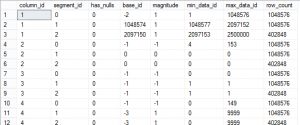From the picture on the left you should be able to see that I have different data in the listed segments and so I can actually execute queries expecting Segment Elimination to take place.

Before advancing to far, the first thing I would love to do is to filter out those Data Types that do not support minimum & maximum values for the segments thus disabling any possibility of the Segment Elimination or Predicate Pushdown. It is easy to determine them, by running the following query, which will filter out all the columns that have only zeros:

```select seg.column_id, col.name, t.name
from sys.column_store_segments seg
inner join sys.partitions part
on part.hobt_id = seg.hobt_id
inner join sys.columns col
on col.object_id = part.object_id and col.column_id = seg.column_id
inner join sys.types t
on col.user_type_id = t.user_type_id
where part.object_id = object_id('dbo.MaxDataTable')
and min_data_id = 0 and max_data_id = 0
group by seg.column_id, col.name, t.name
having count(*) = 3 /* we have 3 segments guaranteed through the number of rows and the MAXDOP = 1 when building Columnstore Index */
order by column_id;
```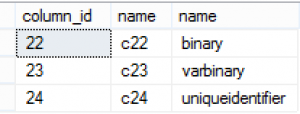You can clearly see that the expectation for the following Data Types (binary, varbinary and uniqueidentifier) is pretty low - even though they have distinct data values, they show through sys.column_store_segments DMV that they do not have any values different to 0.

To ensure that we do have distinct values indeed, you can run the following query, which will give you the number of distinct values for each of the columns:

```select
count(distinct c1),
count(distinct c2),
count(distinct c3),
count(distinct c4),
count(distinct c5),
count(distinct c6),
count(distinct c7),
count(distinct c8),
count(distinct c9),
count(distinct c10),
count(distinct c11),
count(distinct c12),
count(distinct c13),
count(distinct c14),
count(distinct c15),
count(distinct c16),
count(distinct c17),
count(distinct c18),
count(distinct c19),
count(distinct c20),
count(distinct c21),
count(distinct c22),
count(distinct c23),
count(distinct c24)
from dbo.MaxDataTable
```

#### Testing Segment Elimination

There is nothing better as to run a test query and see the practical results for each of the data types, and so I will kick off with the BIGINT data type, which is pretty much the basic requirement of almost any BI or DataWarehouse solution:

```select sum(c1)
from dbo.MaxDataTable
where c1 < 150;
```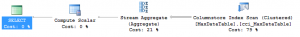This is the execution plan for the above query, you can see that we have only the essential elements with no Filter iterators. The absence of the parallelism iterators (and hence the batch mode if you are wondering) is explained by the low query cost - the whole subtree in my case is estimated to the value of 0.913655.
Should you have any doubts, you can use the column_store_segment_eliminate Extended Event, described in the Clustered Columnstore Indexes – part 47 ("Practical Monitoring with Extended Events")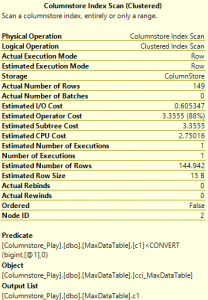You can clearly notice that we have a predicate well defined, it is written under the table of the Columnstore Table Scan - [Columnstore_Play].[dbo].[MaxDataTable].[c1]<CONVERT_IMPLICIT(bigint,[@1],0) . There is no residual predicate and the execution plan does not include any additional filters - everything is fine.

That's fine, we have BigInt with us, but what about the others - numeric for example:
After analysing what's inside the Segments I have created a query that have eliminated the 2nd and the 3rd Segments,

```select sum(c1)
from dbo.MaxDataTable
where c2 > 4. and c2 < 15.
```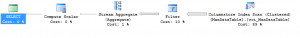If you look at the execution plan, you will notice 2 important things - we are reading too many rows from the Columnstore Table (we are reading them all actually - all 2.5 Million of them) and the new iterator Filter which is actually doing all the work, filtering 69839 rows in my case.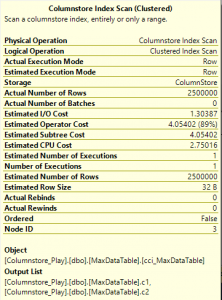In case you are still wondering, there were no events of segment elimination in my Extended Events session which serve as an additional proof that the Numeric values are not supported for Segment Elimination.
This is an interesting situation, showing that the implementation of Segment Elimination for Numeric Data Type is probably on the way, because there is a support for minimum and maximum values in Segment information. I understand that it might be not the biggest priority for the development team because in the most cases the biggest is made with integer data types (they are occupying less space typically and they are used for determining the relationship between fact & dimensions in DWH), but I know that there are a lot of queries where filtering on some numerical information would be a great improvement - filtering out outliers in a IOT table for example :)

Now to the one of the most interesting & probably less useful Data Type - the bit. As you might remember, I have set the column C3 equals to 0 for the first Row Group while the second and the third ones include both 0 and 1 values.
Let's run the query accessing the values of the first Row Group:

```select sum(c1)
from dbo.MaxDataTable
where c3 = 0
```

The actual execution plan includes the very same iterators, as the one with Integer Data Type, with number of values being passed from Columnstore Index Scan to Stream Aggregates being equal to the maximum number of rows in a Row Group - 1048576. Extended Events confirm what is also visible from the execution - that the Segment Elmination took place for this Data Type.

I will avoid going into details of each of the Data Type that I have tested, by simply listing the queries I have run and the respective results:

```
select sum(c1)
from dbo.MaxDataTable
where c1 < 150

select sum(c1)
from dbo.MaxDataTable
where c2 > 4. and c2 < 15.

select sum(c1)
from dbo.MaxDataTable
where c3 = 0

select sum(c1)
from dbo.MaxDataTable
where c4 < 150

select sum(c1)
from dbo.MaxDataTable
where c5 > cast( 150000. as Decimal)

select sum(c1)
from dbo.MaxDataTable
where c6 = 0

select sum(c1)
from dbo.MaxDataTable
where c7 > 150

-- TinyInt
select sum(c1)
from dbo.MaxDataTable
where c8 > 149

-- Money
select sum(c1)
from dbo.MaxDataTable
where c9 >= 150.

-- Float
select sum(c1)
from dbo.MaxDataTable
where c10 > 149.

-- Real
select sum(c1)
from dbo.MaxDataTable
where c11 > 150.

-- Date
select sum(c1)
from dbo.MaxDataTable
where c12 <= dateadd(dd,-1,getDate())

-- DateTimeOffset
select sum(c1)
from dbo.MaxDataTable
where c13 > '1900-04-03 00:00:00.000 +01:00'

-- DateTime2
select sum(c1)
from dbo.MaxDataTable
where c14 >= GetDate()

-- SmallDateTime
select sum(c1)
from dbo.MaxDataTable
where c15 > dateadd(dd,-1,getDate())

-- DateTime
select sum(c1)
from dbo.MaxDataTable
where c16 > dateadd(dd,-1,getDate())

-- Time
select sum(c1)
from dbo.MaxDataTable
where c17 <= '22:42:23.6930000'

-- Char
select sum(c1)
from dbo.MaxDataTable
where c18 > '99.0'

-- Varchar
select sum(c1)
from dbo.MaxDataTable
where c19 > '99.0'

-- nchar
select sum(c1)
from dbo.MaxDataTable
where c20 > N'99.0'

-- nvarchar
select sum(c1)
from dbo.MaxDataTable
where c21 > N'99.0'

-- binary
select sum(c1)
from dbo.MaxDataTable
where c22 < 0x00002D3A57E3CD4CB058BA7D1CA1105C

-- varbinary
select sum(c1)
from dbo.MaxDataTable
where c23 < 0x000019BD1BED5F43A8B9F1BF02433683

-- uniqueidentifier
select sum(c1)
from dbo.MaxDataTable
where c24 < '51F154F1-E5A4-4E09-B77F-00002C4415CA'
```

Here are the results of my investigation on SQL Server 2014:

Data Type Supports Min & Max Predicate Pushdown Segment Elimination 2012 Predicate Pushdown
bigint yes yes yes yes
numeric yes no no type is not supported for precision above 18
bit yes yes yes yes
smallint yes yes yes yes
decimal yes yes yes yes
smallmoney yes yes yes yes
int yes yes yes yes
tinyint yes yes yes yes
money yes yes yes yes
float yes yes yes yes
real yes yes yes yes
date yes yes yes yes
datetimeoffset yes no no type is not supported for precision above 2
datetime2 yes yes yes yes
smalldatetime yes yes yes yes
datetime yes yes yes yes
time yes yes yes yes
char yes no no no
varchar yes no no no
nchar yes no no no
nvarchar yes no no no
binary no no no type is not supported
varbinary no no no type is not supported
uniqueidentifier no no no type is not supported

From the table above we can see that should the Data Type not support the predicate pushdown operation, then obviously there is no Segment Elimination for the Columnstore Indexes and thus the overall performance of the queries should suffer significantly.
The difficulties with the Character Data Types are one of the areas where Columnstore Indexes could improve in the future, since SQL Server 2012 it is known that the performance with those types is a major problem.

Numeric Data Type appears to be one of the 2 major outsiders and as far as I am personally concerned - the biggest one. It should not be completely impossible to solve this one and I would hope that the next SQL Server version shall include support for it.

The surprising situation with DateTimeOffset Data Type is something that is quite unsurprising, since this is not one of the most popular & frequently used Data Types out there. I think that besides focusing on including some of the not supported Data Types such as CLR and (N)Varchar(max) the development team should focus on improving the performance of the existing data types.

to be continued with Clustered Columnstore Indexes – part 50 ("Columnstore IO")

### 15 thoughts on “Clustered Columnstore Indexes – part 49 (“Data Types & Predicate Pushdown”)”

1. mark

Would be nice running these tests on the latest Azure preview as well. They made some CS improvements.

1. Niko Neugebauer Post author

Hi Mark,

I ran the tests on Azure SQLDatabase and at the moment I could not find any improvement for the Data Type support.

Best regards,
Niko Neugebauer

Greetings Niko — Another thorough writeup! One nit: The difference between c2 and c5 in your example is not about decimal vs. numeric (SQL rarely distinguishes between the two), but about declared precision, 18. Same deal with datetimeoffset, where the boundary precision is 2. (P.S. I work for Microsoft).

1. Niko Neugebauer Post author

Hi Vassilis,

thank you very much, your help is truly appreciated!
I have updated the table with your information – wish I had it before SQLBits, where I have presented an updated version of it (mentioning numeric & datetimeoffset precisions but not expanding on the c2 & c5 differences.)

Best regards,
Niko Neugebauer

1. James Lupolt

Hi Niko and Vassilis,

So just to be clear — segment elimination for decimal & numeric types with precision over 18 is currently not supported in SQL 2014 (as of the current release, SP1)?

James

1. Niko Neugebauer Post author

Hi James,

to my understandings, there is no support for Segment Elimination in SQL Server 2014 at all.
It all starts with no support for predicate pushdown (is it coming for SQL Server 2016? :)) for numeric data types, and it does not really matter what precision do you use.

Best regards,
Niko Neugebauer

3. Mato

Hello Niko,

can you explain me the difference between segment elimination and predicate pushdown?

Ty

1. Niko Neugebauer Post author

Hi Mato,

Segment elimination happens when we avoid reading Columnstore Segments and Row Groups, while predicate pushdown is a way of doing data processing at the deep level of the storage engine, without pushing the information into the memory and processing it later. For aggregation or filtering this does miracles.

Best regards,
Niko Neugebauer

4. Alexander Sharovarov

Hi Niko,

Thank you for your great blog post series. I learned a lot from it. We are using Columnstore index for one of our fact tables and I noticed that Aggregate pushdown doesn’t work for float/real data types even though they fit in 8bytes (64bit) size limit. We are running Microsoft SQL Server 2016 (SP1-CU3).

I wonder if I miss something or it is a big. Have you experienced anything like that?

Thank you in advance,
-Alex

1. Niko Neugebauer Post author

Hi Alexander,

thank you for the kind words.
You have written that the data types FIT into 8 bytes Float/Real, but are they declared as such ?
Can you please share the column definition ?

Best regards,
Niko

1. Niko Neugebauer Post author

Hi Alexander,

interesting, I have confirmed that Float & Real are not supported. I thought that there were just other prohibitive limitations for them, but they are a no-go for way too many situations.
I expect the docs to be updated soon.

Regarding Numeric data type, only when it hits 19 precision, will it occupy 9 bytes – the docs are borked again, but before it was well written there, I believe. I have been showing it in my workshops for quite some time.

Best regards,
Niko

5. Suhas

Hi Niko,
Thanks for the post.
When I ran this in SQL 2019, I could see predicate in CCI scan almost all the queries, Too many changes since then and I beileve min and max value influence only segment elimination and not predicate Push down